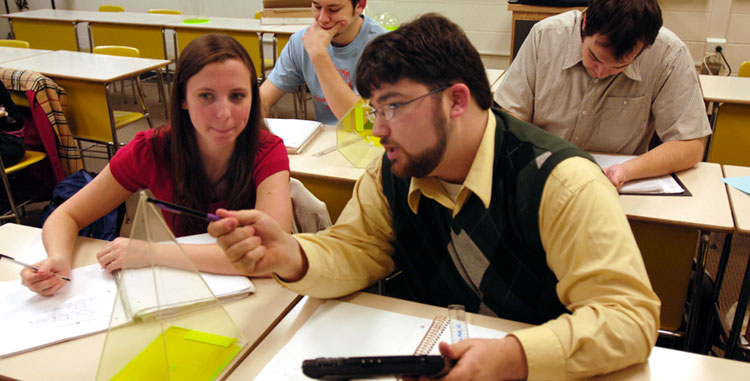# Undergraduate Course Rotation

•## Upper-Level Undergraduate Courses

(Revised fall 2020. Subject to change based on enrollment and budget.)

### Courses Expected to Be Offered Every Semester

• MATH 121 – Calculus I for Natural and Social Sciences
• MATH 125 – Calculus I for Physics, Chemistry, and Mathematics
• MATH 126 – Calculus II for Physics, Chemistry, and Mathematics
• MATH 171 – Introduction to Linear Algebra
• MATH 216 – Probability and Statistics for Natural Sciences
• MATH 225 – Calculus III for Physics, Chemistry, and Mathematics
• MATH 271 – Introduction to Mathematical Proof I
• MATH 272 – Introduction to Mathematical Proof II
• MATH 309 – Discrete Mathematics
• COSC 110 – Problem Solving and Structured Programming
• FIN 310 – Fundamentals of Finance
• FIN 320 – Corporate Finance

### Courses Expected to Be Offered Every Fall

• MATH 111 – Freshman Seminar
• MATH 341 – Differential Equations
• MATH 353 – Theory of Numbers
• MATH 363 – Mathematical Statistics I
• MATH 411 – Univariate Data Analysis
• MATH 413 – Methods of Teaching Mathematics
• EDUC 342 – Pre-Student Teaching Experience II

### Courses Expected to Be Offered Every Spring

• MATH/COSC 343 – Introduction to Numerical Methods*
• MATH 342 – Advanced Mathematics for Applications
• MATH 350 – History of Mathematics
• MATH 355 – Foundations of Geometry
• MATH 412 – Multivariate Statistics
• MATH 480 – Senior Seminar
• EDUC 242 – Pre-Student Teaching Experience I
• EDUC 441 – Student Teaching

* Alternates as MATH and as COSC each spring semester.

## Courses in Rotation

### Fall AY 2020–21

• MATH 421 – Advanced Calculus I
• MATH 446 – Probabilistic Models in O.R.
• MATH 447 – Modeling and Simulation
• MATH 456 – Geometry for Mathematics Instruction
• MATH 458 – Logic and Logical Games for E/M Teachers

### Spring AY 2020–21

• MATH 371 – Linear Algebra
• MATH 420 – Patterns and Functions for E/M Teachers
• MATH 422 – Advanced Calculus II
• MATH 450 – Topics in Applied Computational Mathematics

### Fall AY 2021–22

• MATH 427 – Introduction to Topology
• MATH 448 – Financial Mathematics
• MATH 460 – Technology in Mathematics Instruction
• MATH 471 – Algebra for Mathematics Instruction
• MATH 476 – Abstract Algebra I

### Spring AY 2021–22

• MATH 317 – Probability and Statistics for Mathematics Instruction
• MATH 364 – Mathematical Statistics II
• MATH 416 – Time Series Analysis
• MATH 423 – Complex Variables I
• MATH 445 – Programming Models in O.R.
• MATH 457 – Number Theory for Mathematics Instruction
• MATH 477 – Abstract Algebra II

### Fall AY 2022–23

• MATH 421 – Advanced Calculus I
• MATH 446 – Probabilistic Models in O.R.
• MATH 447 – Modeling and Simulation
• MATH 456 – Geometry for Mathematics Instruction

### Spring 2022–23

• MATH 422 – Advanced Calculus II
• MATH 371 – Linear Algebra
• MATH 450 – Topics in Applied Computational Mathematics
• MATH 461 – Discrete Mathematics for Mathematics Instruction

## Rotation Summaries

• MATH 476/477 and MATH 421/422 alternate years
• MATH 363 every fall/MATH 364 every other spring and MATH 447/450 alternate years
• MATH 445 is offered with MATH 364 and 446 is the semester afterward
• MATH 427 is offered every other fall semester
• MATH 371 and 423 alternate spring semesters
• MATH 448 is offered every other fall semester
• MATH 416 is offered every other spring semester
• MATH 456 and MATH 458 offered every even AY fall
• MATH 317 and MATH 459 offered every even AY spring
• MATH 457 and MATH 471 offered every odd AY fall
• MATH 420 and MATH 461 offered every odd AY spring